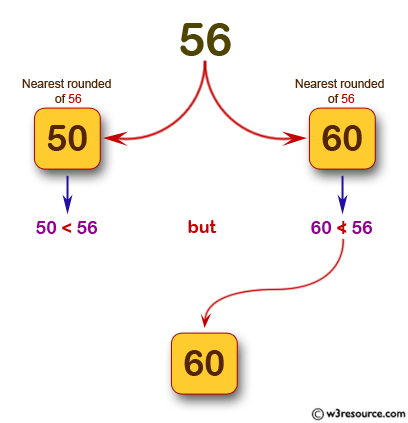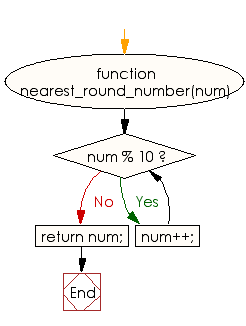# JavaScript: Find the smallest round number that is not less than a given value

## JavaScript Basic: Exercise-128 with Solution

Write a JavaScript program to find the smallest round number that is not less than a given value.

Note: A round number is informally considered to be an integer that ends with one or more zeros. So, 590 is rounder than 592, but 590 is less round than 600.

Pictorial Presentation:Sample Solution:

HTML Code:

``````<!DOCTYPE html>
<html>
<meta charset="utf-8">
<meta name="viewport" content="width=device-width">
<title> Find the smallest round number that is not less than a given value</title>
<body>

</body>
</html>
```
```

JavaScript Code:

``````function nearest_round_number(num) {
while (num % 10) {
num++;
}
return num;
}

console.log(nearest_round_number(56));
console.log(nearest_round_number(592));
``````

Sample Output:

```60
600
```

Flowchart:ES6 Version:

``````function nearest_round_number(num) {
while (num % 10) {
num++;
}
return num;
}

console.log(nearest_round_number(56));
console.log(nearest_round_number(592));
``````

Live Demo:

See the Pen javascript-basic-exercise-128 by w3resource (@w3resource) on CodePen.

Improve this sample solution and post your code through Disqus

What is the difficulty level of this exercise?

Test your Programming skills with w3resource's quiz.

﻿

## JavaScript: Tips of the Day

Returns the sum of the powers of all the numbers from start to end (both inclusive)

Example:

```const sumPower = (end, power = 2, start = 1) =>
Array(end + 1 - start)
.fill(0)
.map((x, i) => (i + start) ** power)
.reduce((a, b) => a + b, 0);
console.log(sumPower(10)); // 385
console.log(sumPower(10, 3)); // 3025
console.log(sumPower(10, 3, 5)); // 2925
```

Output:

```385
3025
2925
```